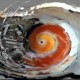# Example: Gromov-Hausdorff distances and the Heisenberg group, PART 3

This post continues the previous one “Gromov-Hausdorff distances and the Heisenberg group, PART 2“.

We have seen that small enough balls in physicist’ Heisenberg group$G$ are like balls in the mathematician’ Heisenberg group$H(1)$ and big balls in$G$ become more and more alike (asymptotically the same) as balls in the euclidean vector space$\mathbb{R}^{2}$.

What is causing this?

Could it be the choice of an euclidean norm on the generating set$D = \mathbb{R}^{2} \times \left\{ 1 \right\}$? I don’t think so, here is why. Let us take any (vector space) norm on$\mathbb{R}^{2}$, instead of an euclidean one. We may repeat all the construction and the final outcome would be: same for small balls, big balls become asymptotically alike to balls in$\mathbb{R}^{2}$ with the chosen norm. The algebraic structure of the limits in the infinitesimally small or infinitely big is the same.

Remember that the group norm is introduced only to estimate quantitatively how the set$D$ generates the group$G$, so the initial choice of the norm is a kind of a gauge.

Could it be then the algebraic structure (the group operation and choice of the generating set)? Yes, but there is much flexibility here.

Instead of$G = \mathbb{R}^{2} \times S^{1}$ with the given group operation, we may take any contact manifold structure over the set$G$ (technically we may take any symplectic structure over$\mathbb{R}^{2}$ and then contactify it (with the fiber$S^{1}$). Sounds familiar? Yes, indeed, this is a step in the recipe of geometric quantization. (If you really want to understand what is happening, then you should go and read Souriau).

Briefly said, put a norm on the kernel of the contact form and declare all directions in this kernel as horizontal, then repeat the construction of the sub-riemannian distance and metric profiles. What you get is this: small balls become asymptotically like balls in the mathematician’ Heisenberg group, big balls are alike balls in a normed vector space.

Therefore, it is not the algebraic structure per se which creates the phenomenon, but the “infinitesimal structure”. This will be treated in a later posting, but before this let me mention an amazing phenomenon.

We are again in the group$G$ and we want to make a map of the small (i.e. of a small enough ball in$G$) into the big (that is into a ball in the vector space$\mathbb{R}^{2}$, which is the asymptotically big model of balls from$G$). Our macroscopic lab is in the asymptotically big, while the phenomenon happens in the small.

A good map is a bi-lipschitz one (it respects the “gauges”, the group norm) from a ball in the vector space$\mathbb{R}^{2}$ to a ball in the Heisenberg group$H(1)$. Surprise: there is no such map! The reason is subtle, basically the same reason as the one which leads to the algebraic structure of the infinitesimally small or infinitely large balls.

However, there are plenty of bi-lipschitz maps from a curve in the ball from the lab (one dimensional submanifold of the symplectic$\mathbb{R}^{2}$, this are the lagrangian submanifolds in this case) to the small ball where the phenomenon happens. This is like: you can measure the position, or the momentum, but not both…

If there are not good bi-lipschitz maps, then there are surely quasi-isometric maps . Their accuracy is bounded by the Gromov-Hausdorff distance between big balls and small balls, as explained in this pedagogical Maps of metric spaces.

## 5 thoughts on “Example: Gromov-Hausdorff distances and the Heisenberg group, PART 3”

1.Stephen KIng says:

This relationship between big and small balls has the same flavor as the S-duality of string theory and QFT! See: http://en.wikipedia.org/wiki/S-duality Dilations seem to have the same effects as the running of the coupling constants in the QFTs…

1.chorasimilarity says:

Thank you for the remark and the link, g and 1/g… I shall look into it, although I am rather ignorant in the string matters.